New data assimilation system DNDAS for high-dimensional models
Huang Qun-bo1, 2, 3, †,, Cao Xiao-qun1, 3, Zhu Meng-bin1, 3, Zhang Wei-min1, 3, Liu Bai-nian1, 3
College of Computer, National University of Defense Technology, Changsha 410073, China
Weather Center of PLA Air Force, Beijing 100843, China
Academy of Ocean Science and Engineering, National University of Defense Technology, Changsha 410073, China

† Corresponding author. E-mail: hqb09@163.com

Project supported by the National Natural Science Foundation of China (Grant Nos. 41475094 and 41375113).

Abstract
Abstract

The tangent linear (TL) models and adjoint (AD) models have brought great difficulties for the development of variational data assimilation system. It might be impossible to develop them perfectly without great efforts, either by hand, or by automatic differentiation tools. In order to break these limitations, a new data assimilation system, dual-number data assimilation system (DNDAS), is designed based on the dual-number automatic differentiation principles. We investigate the performance of DNDAS with two different optimization schemes and subsequently give a discussion on whether DNDAS is appropriate for high-dimensional forecast models. The new data assimilation system can avoid the complicated reverse integration of the adjoint model, and it only needs the forward integration in the dual-number space to obtain the cost function and its gradient vector concurrently. To verify the correctness and effectiveness of DNDAS, we implemented DNDAS on a simple ordinary differential model and the Lorenz-63 model with different optimization methods. We then concentrate on the adaptability of DNDAS to the Lorenz-96 model with high-dimensional state variables. The results indicate that whether the system is simple or nonlinear, DNDAS can accurately reconstruct the initial condition for the forecast model and has a strong anti-noise characteristic. Given adequate computing resource, the quasi-Newton optimization method performs better than the conjugate gradient method in DNDAS.

1. Introduction

Clifford proposed the dual-number theory in 1873, Yang et al. and Denavit extended the theory. Currently, the dual-number theory is mainly applied in the spatial mechanisms, robot design, matrix mechanics, and spacecraft pilot. Li pointed out the importance of the theory in the spatial mechanisms and robot design. Zhang described the displacement relationship in the spatial mechanisms using the dual-number conception. Wang et al. estimated the rotational motion and the translational motion of the chaser spacecraft relative to the target spacecraft based on the dual-number. Hrdina et al. showed that the dual-number is a proper tool for directly classifying the geometric errors and integrating the multiaxis machines’ error models. The dual-number method is similar to the complex-step approximation; Cao et al. applied the complex-variable method to the data assimilation problems of general nonlinear dynamical systems, and also verified the availability for non-differentiable forecast models. However, the complex-step approximation can only be valid for one variable once, which would lead to very expensive cost for large-scale calculation problems. Yu et al. implemented a general-purpose tool to automatically differentiate the forward model using the arithmetic of dual-numbers, which is more accurate and efficient than the popular complex-step approximation, and the computed derivatives are the same as the analytical results up to machine precision. Andrews utilized dual-number automatic differentiation to analyze the local sensitivities of three physical models. The dual-number may serve as a means to simplify a model or focus future model developments and associated experiments. Fike developed the mathematical properties of hyper-dual numbers and then applied the hyper-dual numbers to a multi-objective optimization method. On this basis, Tanaka et al. proposed a method referred to as hyper-dual step derivative (HDSD), which is based on the numerical calculation of strain energy derivatives using hyper-dual numbers, does not suffer from either roundoff errors or truncation errors, and is thereby a highly accurate method with high stability. Recently, Cao et al. formally introduced the dual-number theory into the community of data assimilation in numerical weather prediction (NWP), and proposed a new data assimilation method.

Variational principles for various differential equations have been investigated for centuries. Currently, the most popular data assimilation methods are 3/4D-Var and Kalman filter. The aim of data assimilation is to provide accurate initial state information to the discrete partial differential equations controlling the motions of atmosphere or ocean. A data assimilation system optimizes the combination of the observation data and the prior information, and hence provides an optimum estimation of the initial atmospheric state to the NWP model. Taking the incremental 4D-Var as an example, it measures the distances between the analysis states and observations and also the background states. The cost function can be minimized in terms of increments by an optimization algorithm if the first-order derivatives or gradient of the cost function are calculated and provided. Due to the high non-linearity of the atmospheric models and observation operators in NWP, the tangent linear and adjoint techniques are widely applied in the field of data assimilation. The TL model is the first-order linearized approximation of the forecast model with respect to the background trajectory, which is used for computing the incremental updates with perturbations to the initial state variables. The TL model approximately calculates the perturbed model trajectory, then the cost function can be attained. The gradient of the cost function with respect to the initial condition variable is computed by a backward integration of the AD model. Our ultimate goal is to find out the optimal initial condition by an optimization algorithm, of which the gradient of the cost function is very necessary and important input information.

Constructing the TL and the AD models manually is quite complicated and time-consuming, we should first write out the TL model corresponding to the forward forecast model, then construct the AD model. In order to reduce the difficulty of development, the whole forecast model is usually divided into many small segments, and then we write out the TL and the AD models of each segment line by line. Although using this approach makes the development process much simpler, it is still very easy to make mistakes. Furthermore, the actual atmospheric motion equations are very complicated and need some smoothing processes, such as temporal, spatial, and boundary smoothing. These processes will bring great difficulties to us in developing the AD model codes, and sometimes the constructed AD model cannot be completely reversible for the forward integration model.

Because of the drawbacks described above, now a lot of automatic differentiation tools have been developed, such as TAMC and TAPENADE. Automatic differentiation is possibly implemented by the strict rules of tangent linear and adjoint coding. But there are still some unresolved issues. (i) It is still not a black box tool. (ii) It cannot handle non-differentiable instructions. (iii) It is necessary to create huge arrays to store the trajectory. (iv) The codes often need to be cleaned-up and optimized. (v) It has a worse behavior to second- or higher-order derivatives. At present, generating the AD model is not yet fully automatic in reverse mode. It seems that in the foreseeable future, human intervention will be required to finish the most work of AD in reverse mode.

The optimization algorithms play a very important role in the variational data assimilation systems. There are two series of classical optimizing algorithms: gradient-type method and Newton-type method. The gradient-type method (such as conjugate gradient method, CG) requires only the first-order derivative information, which can be directly employed to large-scale unconstrained optimization problems. But for a non-quadratic cost function, the performance of the CG method deteriorates sharply accompanied by a slow convergence speed. The Newton-type method minimizes the cost function by using the information of the second-order Hessian matrix, and the convergence speed is generally faster than the gradient-type method. In practice, the Hessian of most cost functions are not positive definite symmetric matrices which cannot be represented by an analytical solution, leading to using only the first-order derivative of the cost function (which is called as quasi-Newton method). So far, the BFGS is generally thought to be the best quasi-Newton method which can keep the algorithmic global convergence even if it only uses the one-dimensional inexact searching.

At each iteration of the optimization calculation, it inevitably involves solving and saving the first-order and the second-order Hessian matrix information of the cost function. Furthermore, the dimension of the atmospheric state variables can be up to 108–109 in the data assimilation system. Generally, high-dimensional models have the natural attribute of high nonlinearity, and the majority of the geoscientific systems exhibit strongly non-linear characteristics which are also known as “the curse of dimensionality”. Currently, the linearization numerical approximation schemes are frequently-used in NWP for high-dimensional data assimilation, these approaches would give rise to high resolution regional forecast departure occurring owing to the convergence problems and the calculation accuracy problems. There is a corresponding relationship between the adjoint space and the dual-number space derived from the original space in mathematics. They are equivalent to the adjoint state vector and the model state vector. The dual-number operational rules applied in this paper are strictly without any assumptions and approximations, the convergence and precision of the dual-number method can meet the computing requirements of high-dimensional models. How to quickly and accurately calculate the cost function and its gradient information by optimization algorithms is an important question. Nonlinear differential equations generally do not have analytical solutions, which could be replaced by numerical approximate solutions. However, certain characteristics of the equations tend to be ignored because of the machine truncation error. The most attractive feature of DNDAS is that it can efficiently and accurately calculate the first-order derivative information without TL and AD models.

In this paper, we design an attractive new data assimilation system, dual-number data assimilation system (DNDAS), based on the dual-number automatic differentiation principles. DNDAS can accurately determine the cost function and its gradient without developing TL and AD models. The structure of this paper is organized as follows. The following section introduces the dual-number automatic differentiation theory. Section 3 presents the designed architecture of the DNDAS. Section 4 discusses two optimization algorithms. Section 5 analyzes the results of the simulated data assimilation experiments. Finally, conclusions and plans for future work are given in Section 6.

2. Dual-number automatic differentiation principles

A dual-number can be expressed as a pair of ordered real numbers x and x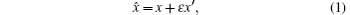where x is the real unit, x′ is the dual unit, and ε is the dual mark which is defined as ε2 = ε3 = ⋯ = 0, ε ≠ 0. To facilitate the representation, equation (1) can be written as = ⟨x,x′⟩. The basic algorithms of dual-number are detailed in Ref. . Here we focus on the principles of dual-number automatic differentiation.

The dual-number function is expanded into Taylor series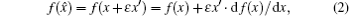thus the function argument can be extended to the dual-number vector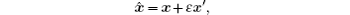then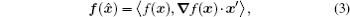where = (1,2,…,n) and x, x′ are n-dimensional control variables.

To obtain the derivation of arbitrary function f (x) at point x0, we calculate the function value f (⟨x0,x′⟩) at the dual-number point x0 in the dual-number space, which equals to evaluating ⟨f (x), f (x) · x′⟩.

In order to compute cost function J(x) and its gradient in DNDAS, we construct the corresponding dual-number vector = ⟨ xi, ei⟩ (i = 1,…,n, ei is the unit vector whose i-th element equals to 1). We then substitute the dual-number vector into the cost function, and the cost function J(x) becomes the dual-number function (). After doing the Taylor expansion, () becomes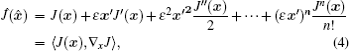where εn = 0(n > 1). We eliminate the component whose order is higher than two. The real and the dual units of () represent the cost function and its gradient, respectively. As shown in Eq. (4), when calculating the first-order derivative of the cost function with respect to the control variables, we just need to perform forward integration once in the dual-number space.

3. DNDAS

In variational data assimilation, the cost function is usually defined as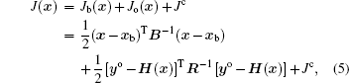where Jb(x) is the background term, Jo(x) is the observation term, Jc describes the physical and dynamic constraint conditions which is optional, x is the control variable vector, xb is the background vector (the prior information), yo is the observation vector, and B and R are the background error covariance matrix and the observation error covariance matrix, respectively. It is seen that the smaller the cost function is, the closer the numerical model solution is to the true atmospheric state. Figures 1 and 2 show the frame of the DNADS and the process of minimization, respectively.

 Figure Option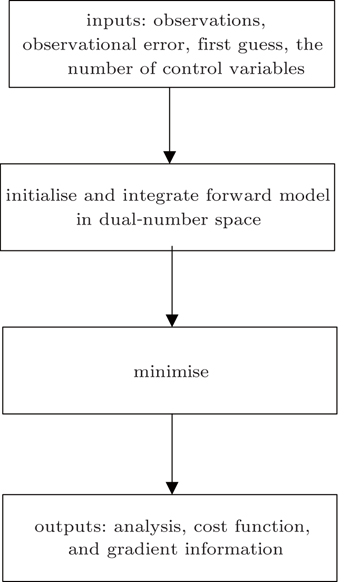Fig. 1. Frame of DNADS.
 Figure Option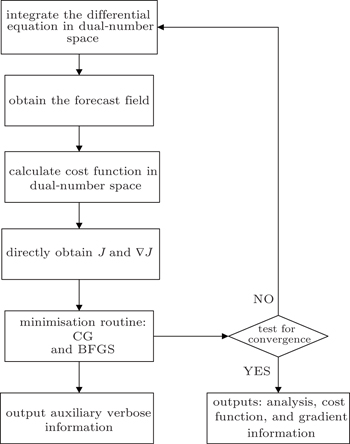Fig. 2. Minimise and output.

Note that, the convergence criterion ∥∇xiJ∥ − ∥∇xi+1J∥ < 10−6 (where ∥·∥ gives the L2 norm) is used to reduce the amount of calculation, and to guarantee that the calculation error is smaller than the machine error. Due to the fact that DNDAS is still in testing phase, we consider the forecast model to be perfect and do not impose the background error in assimilation experiments (Section 5).

4. Optimization algorithms

After obtaining the cost function and its gradient vector, a descent algorithm is chosen to determine the optimal initial state of the forecast model. Optimization or minimization problems have the general form: min f (x), x = (x1,…,xn) ∈ Rn, x represents the control variables, and n is the number of control variables. f : RnR is the cost function which is required to be continuously differentiable. The general process of optimization calculation is defined as follows.

Step 1 Set an initial value x0Rn.

Step 2 Calculate the search direction dk of cost function f at point xk.

Step 3 Calculate the step length factor αk by linear search. If f (xk+1) ≤ f (xk), generate a sequence {xk} of control variables x, namely, xk+1 = xk + αkdk.

Step 4 If the convergence criterion ∥∇f (xk+1)∥ ≤ ε is satisfied, then stop. Otherwise, go back to Step 2.

In this paper, the conjugate gradient algorithm is the Beale restarted memoryless variable metric algorithm. The quasi-Newton algorithm is the BFGS variable metric method. For convenience, the conjugate gradient method is called CG, the BFGS variable metric method is called BFGS. CG and BFGS both use the Davidon cubic interpolation as the basic linear search method to find the best step length. During the calculation process, the step length α must obey the Wolfe criteria, which are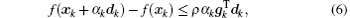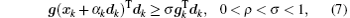where ρ = 0.0001 and σ = 0.9 in this paper. The convergence criterion is ∥g∥ < ε max (1,∥x∥), ε = 10−6.

The steepest descent direction selected by CG has the feature of conjugacy, so only the first-order derivative information is required during the calculation. The algorithm is described as follows.

Step 1 Set the initial point x0 and parameters ρ, σ, ε.

Step 2 Set k = 0, and then calculate f (x0) and g0 = g(x0) = ∇f (x0). Meanwhile, set d0 = −g0 and α0 = 1/∥g0∥.

Step 3 After the initial state is calculated, we need to check whether the minimum is achieved at this point. If ∥g0∥ < ε max(1,∥x0∥), then stop the iteration, output x* = x0. Otherwise, continue.

Step 4 Calculate the initial αk when non-restart, αk = αk−1dk−12/∥dk2. On this basis, make the cubic interpolation to find αk, the termination condition of linear search is the Wolfe criterion, so that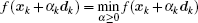.

Step 5 Set xk+1 = xk + αkdk.

Step 6 Compute f (xk+1), g(xk+1), sk = xk+1xk, and yk = g(xk+1) − g(xk).

Step 7 If ∥g∥ < ε max (1,∥x∥), then stop the iteration. Otherwise, continue.

Step 8 When the iteration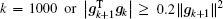, perform Beale restart, obtain a new search direction dk+1, k : = k + 1, go back to Step 4. Otherwise, continue.

Step 9 Compute dk+1.

Step 10 Self-adjustment search direction dk+1,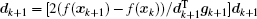, k : = k + 1, go back to Step 4.

4.2. BFGS variable metric algorithm method

The BFGS method requires the cost function to be convex and twice continuously differentiable. The iterative solution can be written as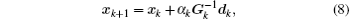where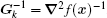. We have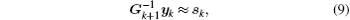where sk = xk+1xk and yk = gk+1gk = ∇f (xk+1) − ∇f (xk).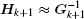is the approximately inverse Hessian matrix of f at xk+1. It can be found from quasi-Newton equation Hk+1 yk = sk that Hk+1 is updated accompanied by {sk,yk}, and avoids direct calculation of the second-order Hessian matrix. We obtain the equation xk+1 = xk + αk Hkdk. The BFGS algorithm is described as follows.

Step 1 Start with an estimate x0 and the estimate of the initial inverse Hessian matrix H0 which is usually the identity matrix I.

Step 2 If ∥gk∥ ≤ ε, then stop. Otherwise evaluate dk+1 = − Hk+1 gk+1, and scale the search direction ∥dk+1∥ = ∥sk−1∥, k = 1,…,n which are the previous n iterations.

Step 3 Set xk+1 = xk + αkdk.

Step 4 Evaluate f (xk+1), g(xk+1), sk = xk+1xk, and yk = g(xk+1) − g(xk).

Step 5 If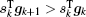and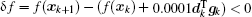, then stop the linear search. Go back to Step 2.

Step 6 Perform the Shanno and Kettler’s extrapolation or Davidon’s cubic interpolation to estimate a new step length αk.

Step 7 Update Hk+1 by the following formula: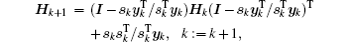go back to Step 2 and repeat the whole process.

The calculation process of BFGS is similar to that of CG, but there are some differences between them. BFGS considers the impact of the scale factor and updates matrix H during the linear search process. It requires more memory resources during the calculation process, i.e., n(n + 7)/2 and 5n + 2, where n is the number of the control variables.

5. Assimilation experiments
5.1. Simple model

Carbon-11 is a radioactive element with a decay rate of 3.46%/min. The attenuation constant is λ = 2.076 s−1. The atomic number density is A0 at t = 0. The atomic number density differential equation is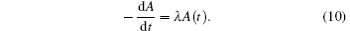The analytical solution is A = A0 eλt.

This first-order ordinary differential equation is treated as a simple dynamic system. Our goal is to assimilate the accurate initial state of the atomic density by using DNDAS.

In the experiment, we integrate Eq. (10) forward by the fourth-order Runge–Kutta method in the real number space, obtaining a numerical integral sequence with the true initial condition A(0) = A0 = 100. The time step length Δt = 0.01, the integral interval is [0, 6], and the assimilation window length is [0, 0.06]. The observations are obtained from the sequence by imposing Gaussian random error N(0, δ), where the error mean is zero and the standard deviation is δ. δ = 0 represents that the observation sequence is noiseless.

The first guess of the atomic density is set as Ab = 8.5. Considering that the observation should be sparse in reality, three observations at 2nd, 4th, and 6th time steps are selected.

Without considering the background information in the experiment (merely considering the observation influence), the discrete form of the cost function is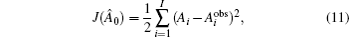where I represents the number of observations, and Ai and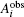are the numerical solution and the observation, respectively.

The dual-number variable corresponding to the initial background variable Ab is 0, 0 = Ab + ε. Then, we perform forward integration in the dual-number space to obtain (), whose real unit is the original cost function J and dual unit is the gradient AJ. Finally, we update the initial state by the optimization algorithm.

Figures 3(a) and 3(b) show the cost function and the gradient norm changing with iterations, respectively. It is seen that the cost function and its gradient norm reach convergence after three iterations, and the accuracy of the gradient norm nearly reaches O(10−8).

 Figure Option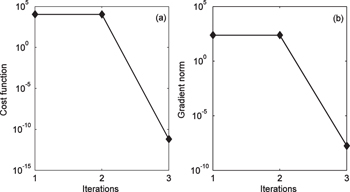Fig. 3. Trends of (a) the cost function and (b) its gradient norm.

Figure 4 shows that the initial state converges to the truth with the increase of iterations, indicating that we obtain an accurate estimation of the unknown initial state by DNDAS. Figure 5(a) shows the comparison between the assimilated forecast trajectory and observations (without observation error). The two trajectories are very close, which illustrates that the assimilated initial value is very accurate for the forecast model. In the meantime, figure 5(b) shows the comparison between the model forecast trajectory (numerical solution) and the true trajectory (analytical solution), which is consistent with the result in Fig. 5(a).

 Figure Option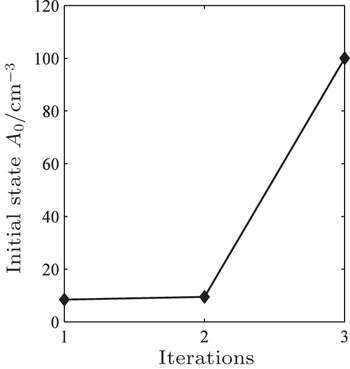Fig. 4. Trend of initial atomic density A0.
 Figure Option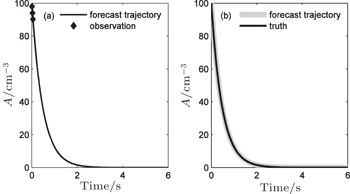Fig. 5. (a) Comparison between the assimilated forecast trajectory and observations, and (b) comparison between the assimilated forecast trajectory and the true trajectory.

However, there are always errors in the observation data, including observation operator errors and so on. Therefore, we consider the validity of DNDAS with Gaussian observational errors δ = 0.1 and δ = 0.2 in the observational sequences, respectively. Table 1 shows that even if there are observational errors, DNDAS still converges to the true value with high precision, indicating that the new assimilation system has a good anti-noise performance.Table 1.

The assimilation results at different observational error levels.

.
5.2. Lorenz-63 chaotic model

Lorenz-63 chaotic system is the most famous nonlinear dynamic system, which is the first example of chaotic dissipative system. The basis of optimal control to the chaotic system is to control the highly sensitive initial state. Therefore, how to obtain the accurate initial conditions is crucial. The Lorenz-63 model equations are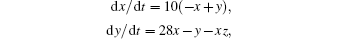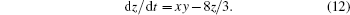We integrate the Lorenz-63 equations forward by the fourth-order Runge–Kutta method in the real number space, with the true initial condition of the model xt = (x0,y0,z0) = (2.0,3.0,2.5), time step length Δt = 0.01, integral interval [0, 1], and assimilation window [0, 0.2]. The observations are obtained by adding Gaussian random error N(0,δ) to the integral sequence of the numerical model.

Set the first guess of the model as xb = (12.0,2.0,9.0). To ensure the effectiveness of the DNDAS, we only select a few observations at 4th, 8th, 12th, 16th, and 20th time steps.

The cost function is defined as follows (without considering the background information):where I represents the number of observations.

The dual-number vector corresponding to the initial background vector xb is 0,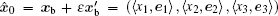, where ei (i = 1,2,3) is the unit vector with the i-th element being 1. We integrate the cost function () in the dual-number space to obtain the original cost function and its gradient (corresponding to the real and the dual units of ()).

The calculation process of the Lorenz-63 model is similar to that discussed in Subsection 5.1, and we will investigate the impact of two different optimization methods on the DNDAS.

For the cost function and its gradient, without observation error (δ = 0), CG and BFGS can quickly reach convergence after 60 iterations and 30 iterations, respectively, and obtain the same accuracy (Fig. 6). The trends of the cost function and its gradient norm are shown in Figs. 6(a) and 6(b). The trends of control variables x, y, and z changing along the iterations are plotted in Figs. 7(a)7(c). We can observe from the plots that the three control variables all converge to the truth by using CG and BFGS, however, the iteration number of BFGS is about half less than that of CG in DNDAS.

 Figure Option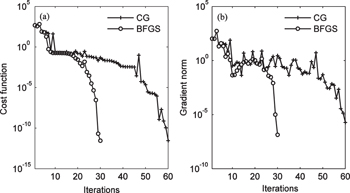Fig. 6. Trends of (a) the cost function and (b) its gradient norm.
 Figure Option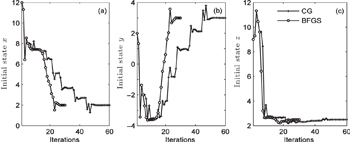Fig. 7. Trends of variables (a) x, (b) y, and z.

To verify the validity of the DNDAS under the existence of observation error, we have done four groups of experiments (experiment groups 1–4) with δ = 0.0, δ = 0.1, δ = 0.2, and δ = 0.5. In each group of experiment, we evaluate and compare the performances of DNDAS (with BFGS and CG methods), 3D-Var, and 4D-Var, wherein 3D-Var and 4D-Var utilize the identical observation conditions and background conditions as used by DNDAS. Table 2 illustrates the results from the DNDAS, it is obvious that no matter of BFGS or CG, the converged values are all close to the true ones at the end of the iterations in DNDAS. Even with δ = 0.5, the accuracy is still high, which indicates that the DNDAS has an anti-noise feature for the nonlinear chaotic model. Departures of the analysis from the truth in experiment groups 2 and 3 are presented in Fig. 8. Because of the limited space, we only present the departures of variables y (Fig. 8(a)) and z (Fig. 8(b)), the trends of variable x is similar (not shown). In experiment group 2, the departures of variables y and z are a little bigger in DNDAS than those in 3D-Var and 4D-Var when the observation error is small. However, the performance of DNDAS is better at higher observation error levels.Table 2.

Assimilation results at different observation error levels.

.
 Figure Option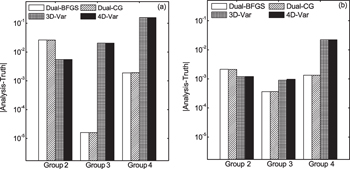Fig. 8. The absolute values of departures (analysis minus truth) of variables (a) y and (b) z in the experiments.

Finally, it is found that, for small-scale problems, the running times of CG and BFGS are nearly the same (see Fig. 9(a)), but the iteration number of BFGS is half less than that of CG (see Fig. 9(b)). The main reason is that CG needs to test two step length factor α cases satisfying the Wolfe criterion during the linear searching, whereas BFGS only needs to test one case. Consequently, CG is suitable for large-scale problems, while BFGS is suitable for small-scale problems which can improve the convergence performance in DNDAS. In addition, with the increase of the observation errors, especially for the 4D-Var method, the running time and the iteration number increase significantly, which further evidences the good anti-noise performance of the DNDAS.

 Figure Option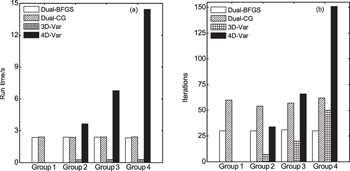Fig. 9. (a) Running time and (b) iterations in the experiments.
5.3. High-dimensional model (Lorenz-96)

The Lorenz-96 model is a dynamical system which was introduced by Lorenz in 1996. The model equations contain quadratic, linear, and constant terms representing advection, dissipation, and external forcing. Numerical integration indicates that small errors (differences between solutions) tend to double in about 2 days. It is defined as follows: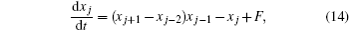where xj (j = 1,…,N) represents the model state which is the values of some atmospheric parameters (such as temperature), and F is a forcing constant which is a common value known to cause a chaotic behavior (we usually set F = 8.0 for maintaining the unbiased feature of the model). There are N control variables. The boundary conditions are set to be x−1 = xN−1, x0 = xN, xN+1 = x1, so the variables xj form a cyclic chain.

We conduct four groups of experiments, the numbers of control variables are 4, 40, 100, and 400 respectively, and two optimization algorithms (CG and BFGS) are both applied in each group; these experiments are named Exp4-CG/BFGS, Exp40-CG/BFGS, Exp100-CG/BFGS, and Exp400-CG/BFGS.

We integrate the Lorenz-96 equations forward 1000 steps with time step length Δt = 0.001 by the fourth-order Runge–Kutta method in the real number space. The true initial condition of the Lorenz-96 model is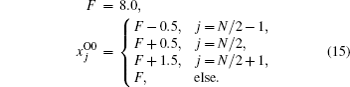The previous 100 integral values are divided into 10 intervals in order. The observations are obtained by taking the last value in each interval. Due to the high precision of the fourth-order Runge–Kutta method, we take observations as unbiased.

The first guess of the forecast model is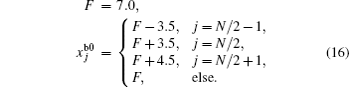The cost function is defined as follows (without considering the background information):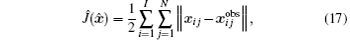where I represents the number of observations.

Subsequently, we construct the dual-number vector as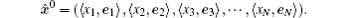The whole calculation process is similar to that described for the Lorenz-63 experiment. Next we provide the results of our assimilation experiments.

5.3.1. The convergence

Figures 10 and 11 show that the cost function and the gradient norm both reach convergence rapidly with the same precision, and the iteration number of the BFGS is about half less than that of the CG in each experiment except for Exp4-CG/BFGS. It can be concluded that, for the forecast model with middle-high dimensional control variables, the convergence performance of the quasi-Newton method is still generally better than the conjugate gradient method in DADAS. Furthermore, there are only small amounts of change and reduction in iterations and calculation accuracy of the cost function and its gradient norm with the increase of the number of control variables. The above results have demonstrated that DNDAS works well, and it is applicable to the high-dimensional variables data assimilation with stability and reliability.

 Figure Option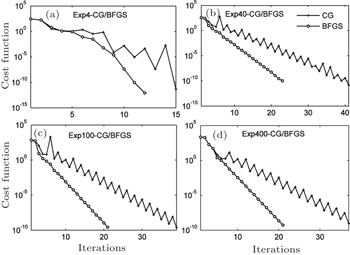Fig. 10. Trends of the cost function: (a) Exp4-CG/BFGS, (b) Exp40-CG/BFGS, (c) Exp100-CG/BFGS, and (d) Exp400-CG/BFGS.
 Figure Option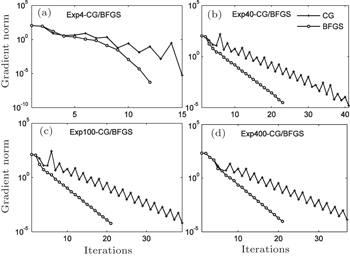Fig. 11. Trends of the gradient norm: (a) Exp4-CG/BFGS, (b) Exp40-CG/BFGS, (c) Exp100-CG/BFGS, and (d) Exp400-CG/BFGS.
5.3.2. The quality of assimilated results

Figure 12(a) shows the analysis increment (analysis minus observation) and observation increment (observation minus background) in the Exp400-BFGS experiment. Comparing with the observation increment (Fig. 12(b)), the magnitude of the analysis increment in Fig. 12(a) reaches a very small scale O(10−6), indicating that the analysis field assimilated by DNDAS is quite close to the truth. Fluctuations of analysis increments in the intermediate zone in Fig. 12(a) also show the correlation among Lorenz-96 model’s control variables because we provide different initial values for these variables. The Exp400-CG experiment has a very similar pattern (not shown).

 Figure Option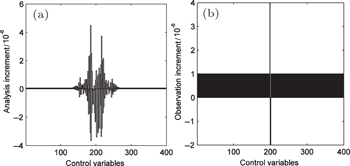Fig. 12. (a) Analysis increment and (b) observation increment of each control variable in Exp400-BF experiment.

In addition, we restart the forecast model with the assimilated analysis filed. Figure 13(a) suggests that the forecast trajectory (broad line) is consistent with the true trajectory (thin line) of the 199th, 200th and 201st control variables. Figure 13(b) shows the consistency between the background trajectory (blue line), the assimilated forecast trajectory (black line), the true trajectory (red line), and the observation (green dot) of the 199th variable. These results also verify the availability for data assimilation by DNDAS.

 Figure Option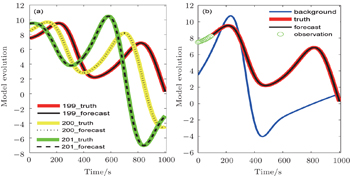Fig. 13. (a) Comparison between the assimilated forecast trajectory and the truth of the 199th, 200th, and 201st control variables. (b) Comparison between the assimilated forecast trajectory, the truth, the observation, and the background trajectory of the 199th control variable.
6. Conclusion and perspectives

The results of this study suggest that the DNDAS works well. Whether it is a simple or a nonlinear chaotic model, the DNDAS can quickly converge to the desired accuracy, indicating that the new assimilation system has a certain universality. The fact that the DNDAS is still competent under the condition of imposing observation error and not introducing background information indicates its robustness. The framework of DNDAS is so simple that it can be coded and transplanted to other computing platforms flexibly.

For DNDAS, the optimization methods (such as CG and BFGS) have a slight influence on the running time. The iteration number of BFGS is about half less than that of CG, however, BFGS needs more memory storage space and can be too expensive for large scale applications. We should do more experiments to determine the most efficient optimization algorithm.

Results in Subsection 5.3 show that the DNDAS is suited for forecast models with middle-high dimensional control variables. Moreover, the convergence speed and the calculation accuracy will not increase with the expansion of the dimension in the control variables.

The DNDAS in the paper is only applicable to calculate the first-order derivatives. If you wish to get the higher-order derivatives, a more advanced theory needs to be explored, such as the hyperdual number theory. The innovative approach might be more suitable for computing the second-order Hessian matrices information in minimization algorithms, bringing about more exact second-order derivatives than the traditional methods. Further benefits generated from the advanced theory may still have to be verified.

In summary, the DNDAS system performs well in high-dimensional forecast models and has potential to become a new data assimilation system. We aim to move to practical application of the DNDAS in the future. It is expected that more advanced optimization algorithms and the hyper-dual number theory will be the next promising tools to improve the DNDAS.

Reference
 1 Clifford W K1871Proceedings of the London Mathematical SocietyLondon: Oxford University Press381 2 Yang A TFreudenstein F 1964 J. Appl. Mech. 31 300 3 Denavit J1956Description and Displacement Analysis of Mechanisms Based on (2x2) Dual Matrices (Ph.D. Dissertation)EvanstonNorthwestern University 4 Li D W1992J. South China Agr. Univ.1388(in Chinese) 5 Zhao T Z1994J. B. Inst. Techno.1450(in Chinese) 6 Wang J YLiang H CSun Z WZhang S J2012Acta Aeronaut. Astronaut. Sin.331881(in Chinese) 7 Hrdina JVasik P 2014 J. Appl. Math. 91 688 8 Cao X QSong J QZhang W MZhao Y LLiu B N2013Acta Phys. Sin.62(in Chinese)170504 9 Yu W BBlair M 2013 Comput. Phys. Commun. 184 1446 10 Andrews J M 2013 J. Fluids Eng. 135 0612061 11 Fike J A2013Multi-objective Optimization using Hyper-dual Numbers (Ph. D. Dissertation) Palo AltoStanford University 12 Tanaka MSasagawa TOmote RFujikawa MBalzani DSchroder J 2015 Comput. Methods Appl. Mech. Engrg. 283 22 13 Cao X QHuang Q BLiu B NZhu M BYu Y2015Acta Phys. Sin.64130502(in Chinese) 14 Cao X QSong J QZhang W MZhao J 2011 Chin. Phys. 20 090401 15 Zhang LHuang S XShen CShi W L 2011 Chin. Phys. 20 0129201 16 Zhang LHuang S XShen CShi W L 2011 Chin. Phys. 20 0119201 17 Zhao G ZYu X JXu YZhu J 2010 Chin. Phys. 19 070203 18 Zhong JFei J FHuang S XDu H D2012Acta Phys. Sin.14149203(in Chinese) 19 Zhang W MCao X QSong J Q2012Acta Phys. Sin.24249202(in Chinese) 20 Zhu M BZhang W MCao X QYu Y 2014 Chin. Phys. 23 069202 21 Zhu M BZhang W MCao X Q2013Acta Phys. Sin.63189203(in Chinese) 22 Cao X QSong J QZhu X QZhang L LZhang W MZhao J 2012 Chin. Phys. 21 020203 23 Leng H ZSong J QCao X QYang J H2012Acta Phys. Sin.61070501(in Chinese) 24 Hu G GGao S SZhong Y MGao B B 2015 Chin. Phys. 24 070202 25 Leng H ZSong J Q 2013 Chin. Phys. 22 030505 26 Liu B NZhang W MCao X QZhao Y LHuang Q BLuo Y2015Chin. J. Geophys. Ch.581526(in Chinese) 27 Benedetti A2014ECMWF Tranning Course 28 Errico M RVukicevic TRaeder K 1993 Tellus 45 462 29 Giering RKaminski T 1998 Trans. Math. Software 24 437 30 Hascoet L2004Proceedings of the ECCOMAS ConferenceJuly 24–28, 2004Jyvaskyla, Finland 31 Griewank A1989Mathematical Programming: Recent Developments and ApplicationsTanabeKluwer Academic83 32 Sambridge MRickwood PRawlinso NSommacal S 2007 Geophys. J. Int. 170 1 33 Zhu X Q2008Ph. D. DissertationChangshaNational University of Defense Technology(in Chinese) 34 Snyder CBengtsson TBickel PAnderson J 2008 Mon. Wea. Rev. 136 4629 35 Wu Q K2012Acta Phys. Sin.61020203(in Chinese) 36 Shanno D FPhua K H 1978 Math. Program 14 149 37 Shanno D FPhua K H 1980 ACM Trans. On Math. Software 6 618 38 Davidon W C 1991 SIAM J. Optimiz. 1 1 39 Wolfe P 1971 SIAM Rev. 23 054203 40 Andrei N2008ICI Technical Report 13/08 41 Shanno D FPhua K H 1976 ACM Trans. Math. Software 2 87 42 Shanno D FKettler P C 1970 Math. Comput. 24 657 43 Lorenz E N 1963 J. Atoms. Sci. 20 130 44 Cao X Q 2013 Acta Phys. Sin. 62 230505 (in Chinese) 45 Lorenz E N1996ECMWF Proc. Seminar1 46 Lorenz E NEmanuel K A 1998 J. Atmos. Sci. 55 399 47 Ott EHunt B RSzunyogh IZimin A VKostelich ECorazza MKalnay EPatil D JYorke J A 2004 Tellus 56 415